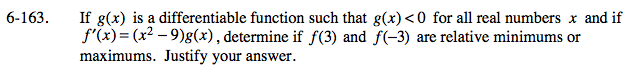### Home > CALC > Chapter 6 > Lesson 6.4.3 > Problem6-163

6-163.Determine if f(3) is an extrema candidate.
That is, does f '(3) = 0 or does f '(3) = DNE

f '(3) = (3²−9)(some negative value) = 0
Yes, f(3) is an extrema candidate! It could be a relative max, a relative min, or neither.

Determine if f(3) is a relative max, relative min or neither. Use the 1st-derivative test:
To do this, determine if f ′(x) changes sign at x = 3.
Evaluate a little to the left and a little to the right.
To the left: f ′(2.9) = (2.9³ − 9)(some negative value) = (negative)(negative) = positive.
To the right: f ′(3.1) = (3.1³ − 9)(some negative value) = (positive)(negative) = negative.
Conclusion: Since f ′(3) = 0 and f ′(x) changes from positive to negative at x = 3, therefore f(3) is a relative maxima.

Now test f(−3).